# Rational Function

Also found in: Dictionary, Wikipedia.

## rational function

[′rash·ən·əl ′fəŋk·shən]
(mathematics)
A function which is a quotient of polynomials.

## Rational Function

a function obtained as a result of a finite number of arithmetic operations (addition, multiplication, and division) on a variable x and arbitrary numbers. A rational function has the form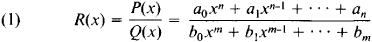where a0a1,…, an and b0, b1,…,bm (a0 ≠ 0, b0 ≠ 0) are constants and η and m are nonnegative integers.

A rational function is defined and continuous for all values of x other than values that are zeros of the denominator Q(x). If x is a zero of multiplicity k of the denominator Q(x) and is simultaneously a zero of multiplicity r (rk) of the numerator P(x), then R(x) has a removable discontinuity at the point x. If, however, r < k, then R(x) has an infinite discontinuity, or pole, at x.

A polynomial is the special case of a rational function where m = 0; polynomials are thus sometimes called rational integral functions. Every rational function is the quotient of two polynomials. A linear fractional function is another example of a rational function.

If in equation (1) n < m (m > 0), then the rational function is said to be proper. If, on the other hand, nm, then R(x) may be represented as a sum of a polynomial M(x) of degree η - m and a proper rational function R1(x) = P1(x)/(Q(x):

R(x) = M(x) + R1(x)

The degree of the polynomial P1 (x) is less than m, and the polynomials M(x) and P1(x) are uniquely determined by the formula

P(x) = M(x)Q(x) + P1(x)

which expresses Euclid’s theorem for polynomials.

It follows from the definition of a rational function that functions obtainable by a finite number of arithmetic operations on rational functions and arbitrary numbers are also rational functions. In particular, a rational function of a rational function is a rational function. A rational function is differentiable at all points at which it is defined, and its derivative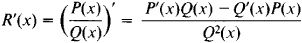is also a rational function.

In accordance with the above, the integral of a rational function reduces to the sum of the integral of a polynomial and the integral of a proper rational function. The integral of a polynomial is a polynomial, and its calculation does not present any difficulty. The following formula for the decomposition of a proper rational function R1(x) into partial fractions is used to calculate the integral of such a function: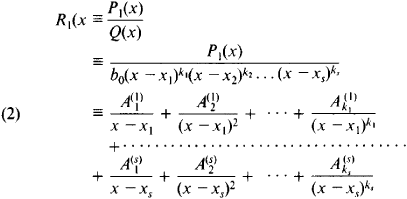Here, x1, …, xs are distinct zeros of the polynomial Q(x) of multiplicity k1, …, ks, respectively (k1 + … + ks = m), and the Aj(i) are constant coefficients. The decomposition (2) of a rational function into partial fractions is unique.

If the coefficients of the polynomials P1(x) and Q(x) are real numbers, the complex zeros of the denominator Q(x). if any, come in conjugate pairs. The partial fractions in the decomposition (2) corresponding to each such pair can be combined into real partial fractions: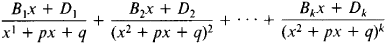where the trinomial x2 + px + q has conjugate complex zeros (4 q > p2). The method of undetermined coefficients may be used to determine the coefficients Aj(i) Bj, and Dj.

The integrals of the partial fractions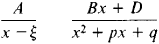are not rational functions: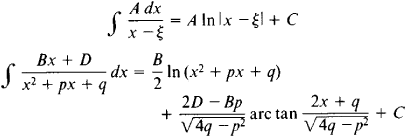The situation is different for the integrals of the partial fractions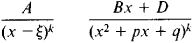where k > 1. The integral of the first is a rational function, and the integral of the second is the sum of a rational function and an integral of the same type as when k = 1. Thus, the integral of any rational function that is not a polynomial can be represented as a sum of rational functions, arc tangents, and logarithmic functions. M. V. Ostrogradskii provided an algebraic method of determining the rational part of an integral of a rational function; his method requires neither decomposition of the rational function into partial fractions nor integration (seeOSTROGRADSKII METHOD).

The rational functions are an extremely important class of elementary functions. Rational functions of several variables are also studied. They are obtained as a result of a finite number of arithmetic operations on their arguments and arbitrary numbers. Thus,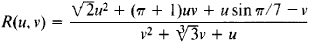is an example of a rational function of the two variables u and v.

Rational functions have come into wide use in the mid-20th century in the approximation of functions (seeAPPROXIMATION AND INTERPOLATION OF FUNCTIONS).

References in periodicals archive ?
It is a theorem of Liouville , reproven later with purely algebraic methods, that for rational functions f and g, g is nonconstant, the antiderivative
In this paper, an extended Newmark-FDTD approach for the complex dispersive medium is presented in terms of polarizability in the form of a quadratic complex rational function, in which the time-stepping equation of the polarization vector is obtained by the Newmark algorithm used widely in finite-element time-domain (FETD) computation.
Recall the above definition of degree of rational function; we have
Lee, "A transformed rational function method and exact solutions to the 3+1 dimensional Jimbo-Miwa equation," Chaos, Solitons & Fractals, vol.
Proposition 5.1 (, Proposition 2.1) The curve [??] over the finite field [F.sub.q] given by the Kummer equation y (r) = [micro](x), where r divides q - 1 and the rational function [mu](x) is not the d-th power of an element [nu] (x) [member of] [F.sub.q] (x) for any divisor d of r with d > 1, it has the following properties:
Lee, "A transformed rational function method and exact solutions to the 3 + 1 dimensional Jimbo-Miwa equation," Chaos, Solitons & Fractals, vol.
After the introduction, the second section presents the analysis of the approximation with a rational function. The third deals with a method of vector fitting.
(3) a Rational Function Approximation (RFA) of the matrices [Q.sub.hh](M, k), and [Q.sub.hg](M, k) is performed.
being symmetric rational function and knowing that 1 has already been proved to have its own valid decomposition, we have:
As an example, due to the symbolic and numeric capabilities of Mathematica, the [ARFact-Scalar] algorithm  always computes the factorization index of the considered non-singular scalar rational function.
We say that a meromorphic function f belongs to the class W if f is an elliptic function, or a rational function of [e.sup.[alpha]z], [alpha] [member of] C, or a rational function of z.

Site: Follow: Share:
Open / Close